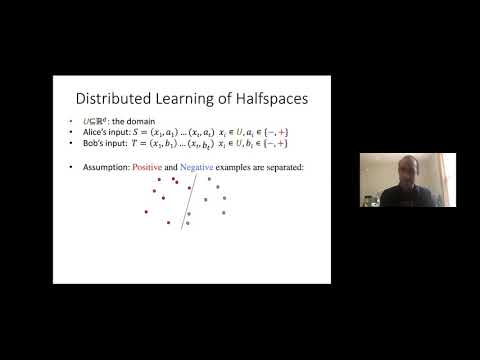## Convex Set Disjointness, Distributed Learning of Halfspaces, and Linear Programming

May 12, 2020
IAS
Presenters: Shay Moran
Length: 2 hours

Watch Video

#### Abstract

Distributed learning protocols are designed to train on distributed data without gathering it all on a single centralized machine, thus contributing to the efficiency of the system and enhancing its privacy. We study a central problem in distributed learning, called Distributed Learning of Halfspaces: let U \subset R^d be a known domain of size n and let h:R^d —> R be an unknown target affine function. A set of examples {(u,b)} is distributed between several parties, where u \in U is a point and b = sign(h(u)) \in {-1, +1} is its label. The parties' goal is to agree, using minimum communication, on a classifier f: U—>{-1,+1} such that f(u)=b for every input example (u,b). (In practice, the finite domain U is defined implicitly by the representation of d-dimensional vectors which is used in the protocol.) We establish a (nearly) tight bound of ~$\Theta$ (d*log n) on the communication complexity of the problem of distributed learning of halfspaces in the two-party setting. Since this problem is closely related to the Convex Set Disjointness problem in communication complexity and the problem of Distributed Linear Programming in distributed optimization, we are able to derive upper and lower bounds of ~O(d^2\log n) and ~Ω(d\log n) for both of these basic problems as well. Our main technical contribution lies in the design and analysis of the protocols which imply the upper bounds. To this end, we introduce a technique called Halfspace Containers, allowing for a compressed approximate representation of every halfspace. Halfspace containers may be of independent interest and are closely related to bracketing numbers in statistics and to hyperplane cuttings in discrete geometry. Joint paper with Mark Braverman, Gillat Kol, and Raghuvansh R Saxena